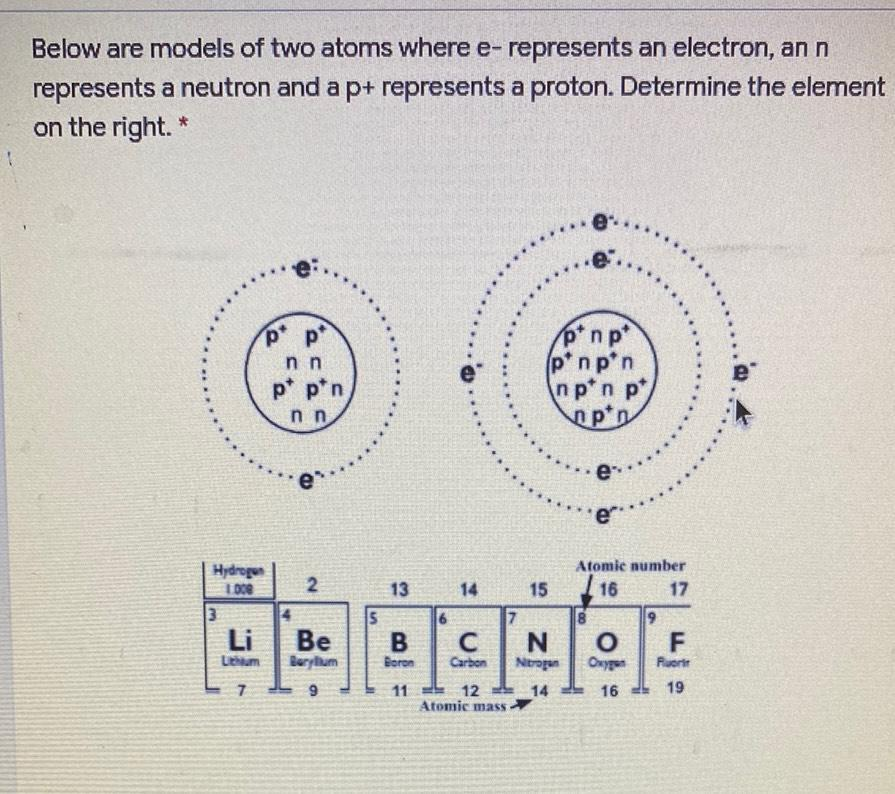Question:

# Below are models of two atoms where e- represents an electron, an n represents a neutron and a p+ represents a proton. DetermineBelow are models of two atoms where e- represents an electron, an n represents a neutron and a p+ represents a proton. Determine the element on the right. * po p* p* np* pinpin npin p pin nn p* pin nn e e. 2 Atomie number 17 I 16 Hydrogen I DE 3 Li Lithium Be Berylum 13 14 15 15 7 B C N Boron Carbon Nitrogen 11 12 14 Atomic mass 8 O Oxygen 16 9 F Fluorit 19 7